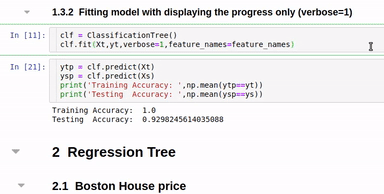Machine Learning From Scratch

• Without using any ML libraries

(Few visualisations are limited to 2D data only, others can be used for any dimenntions)

1. Logistic Regression

Code ans examples are here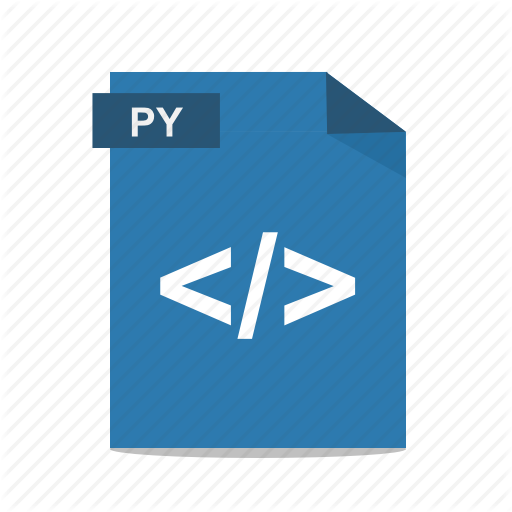``````from LogisticRegression import LR # given code
clf = LR(X,y,alpha=0.003,polyfit=True,degree=5,lambd=2)
fig=plt.figure(figsize=(8,4))
gs=GridSpec(1,2)

for i in range(100):
Clf.fit(X,y,itr=10)
ax1.clear()
Clf.Bplot(ax1,hardbound=False)
ax2.clear()
Clf.LCurvePlot(ax2)
fig.canvas.draw()
plt.pause(0.001)

clf.predict(X)
W,b =clf.getWeight()

``````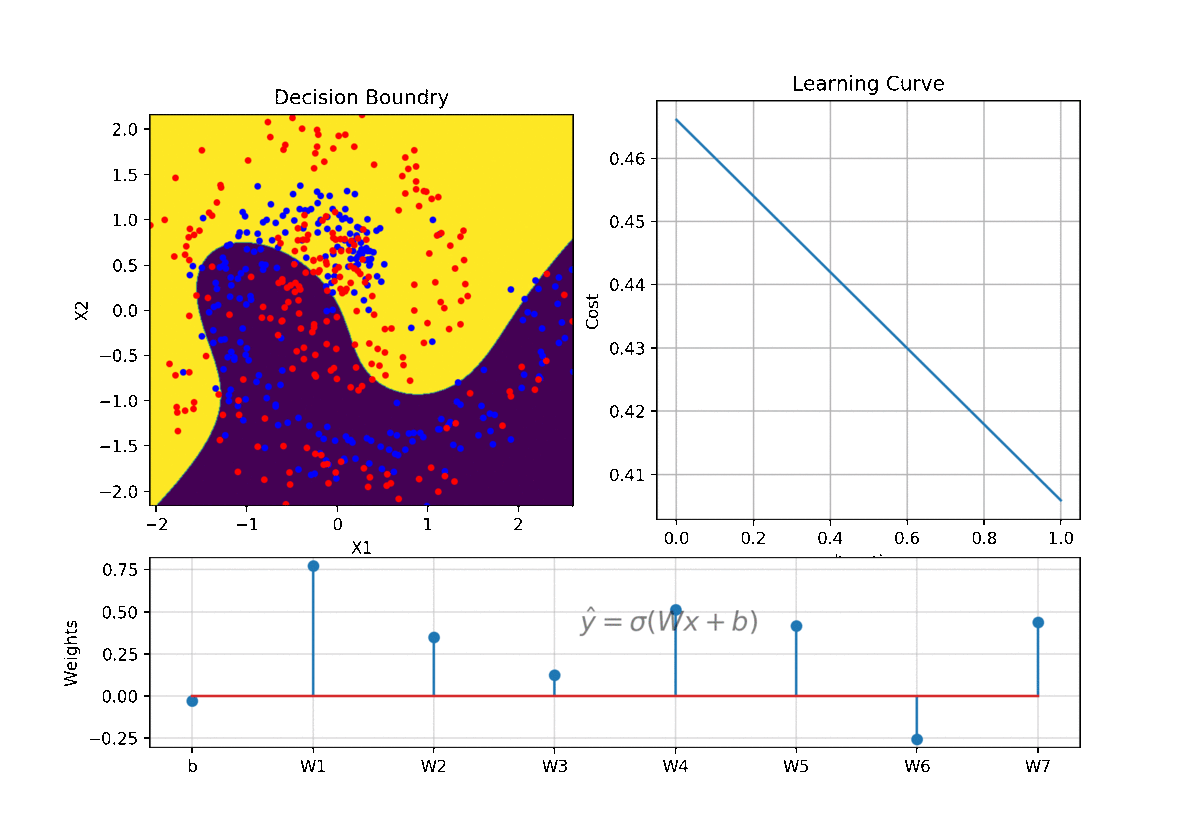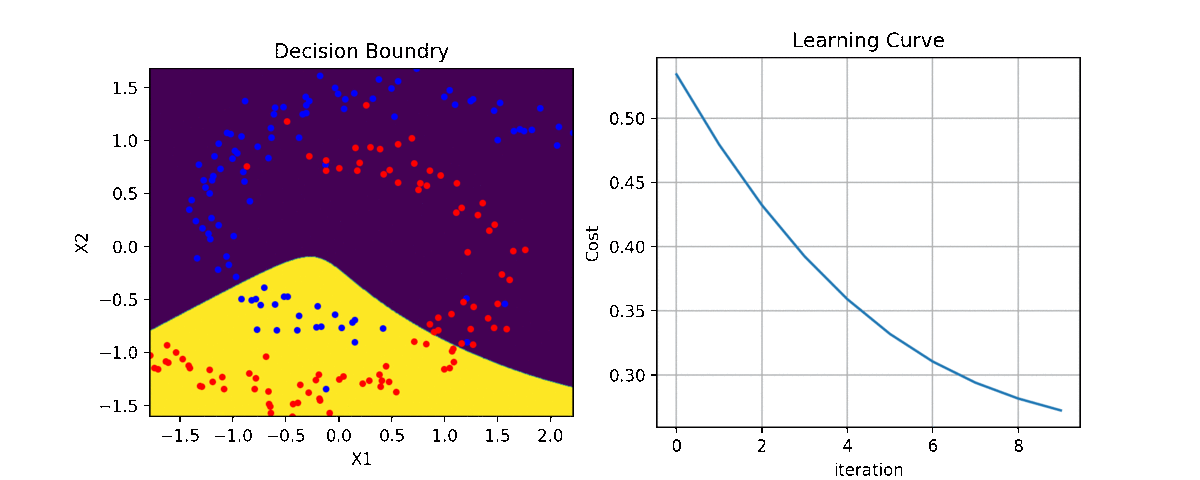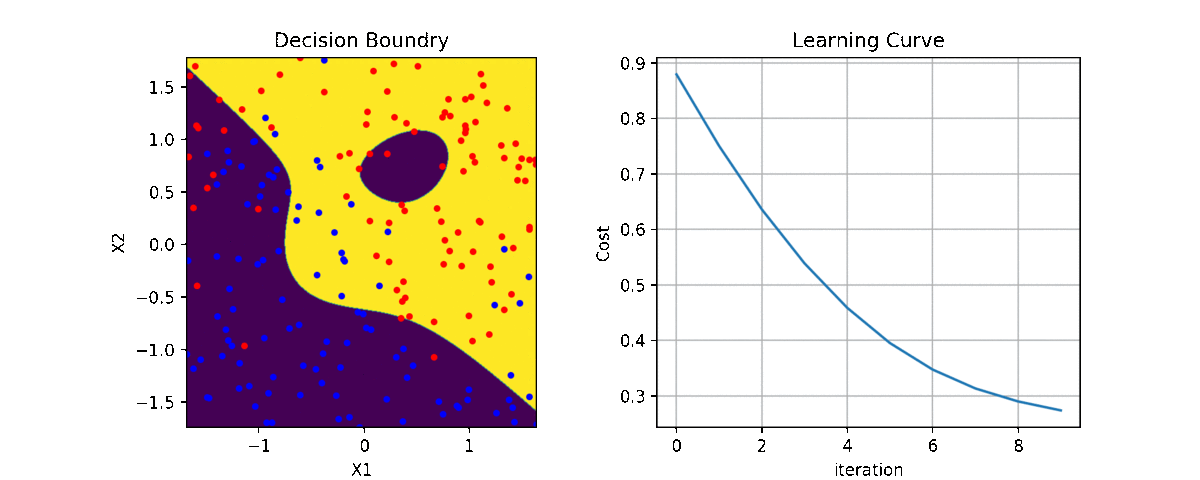2. Deep Neural Network - Deeplearning

Code and examples are here

Full detail of implementation and use of code is describe here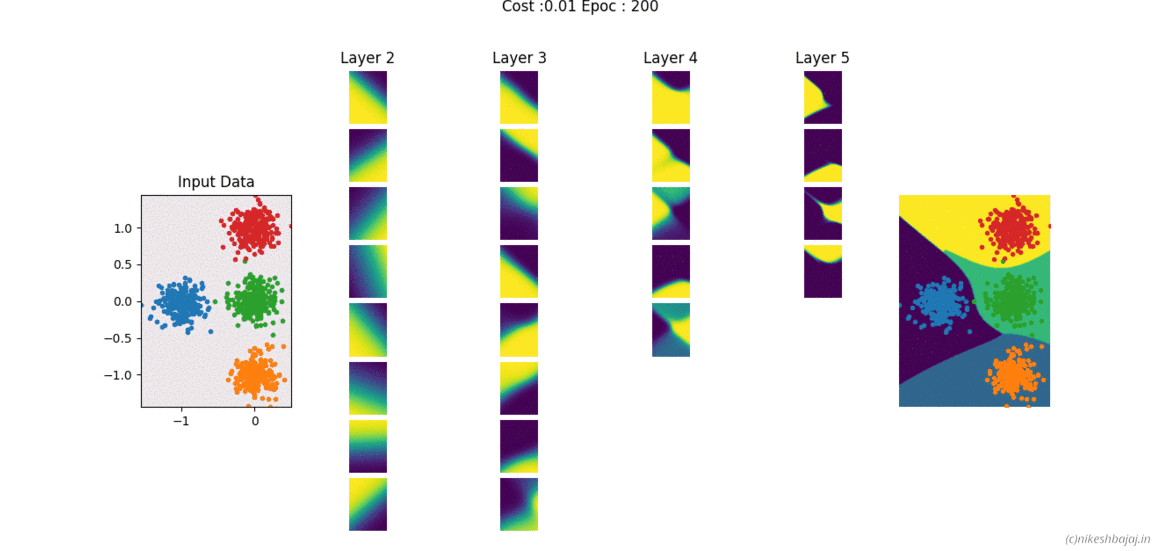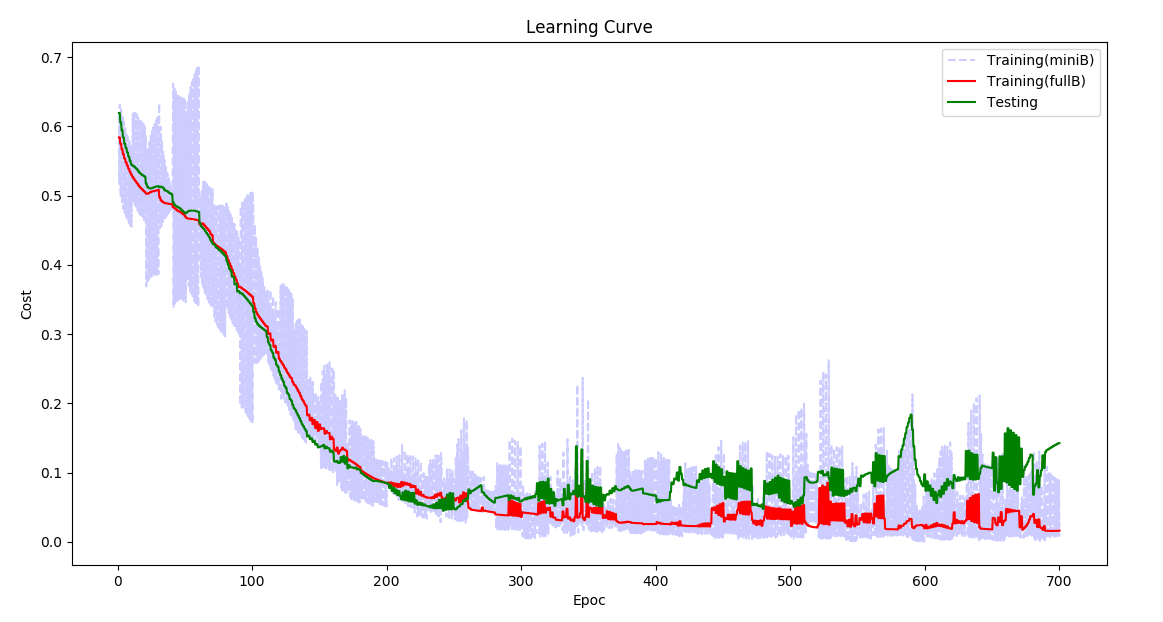3. Neural Network (simple structure-fully connected) with any number of layers (Matlab/Octave)

Code and examples here

Network can be created and trained as for example

``````W= NeuralNet(X,y,HL,Iterations,alpha,verbose);

% For 2 hidden layers with 5 and 3 neurons, 500 iteration and 0.1 alpha(learning rate)
% input and output layers are chosen according to data X,y provided

W= NeuralNet(X,y,[5,3],500,0.1,1);

% for 8 hidden layers
W= NeuralNet(X,y,[15,10,10,10,5,5,5,3],100,0.1,1);

returns weights W of each layer

``````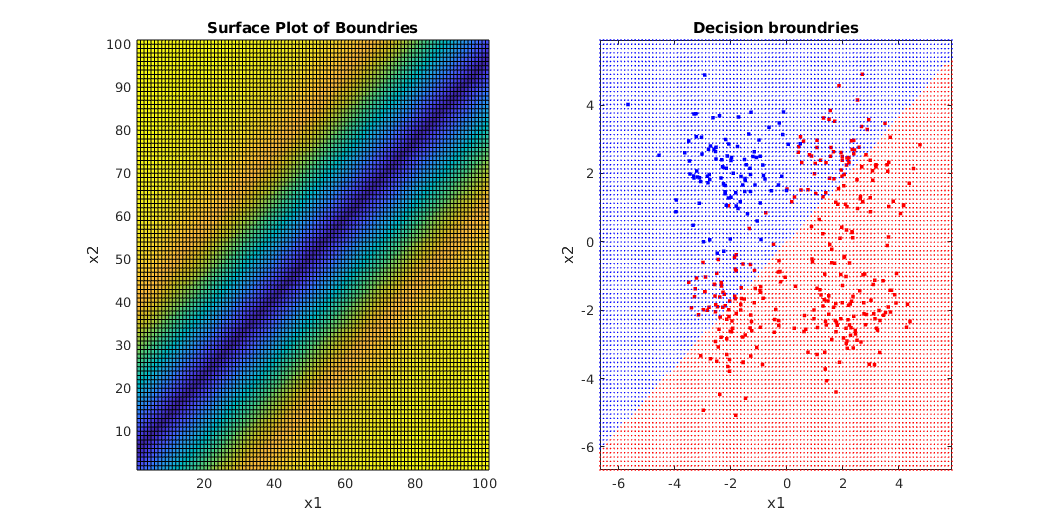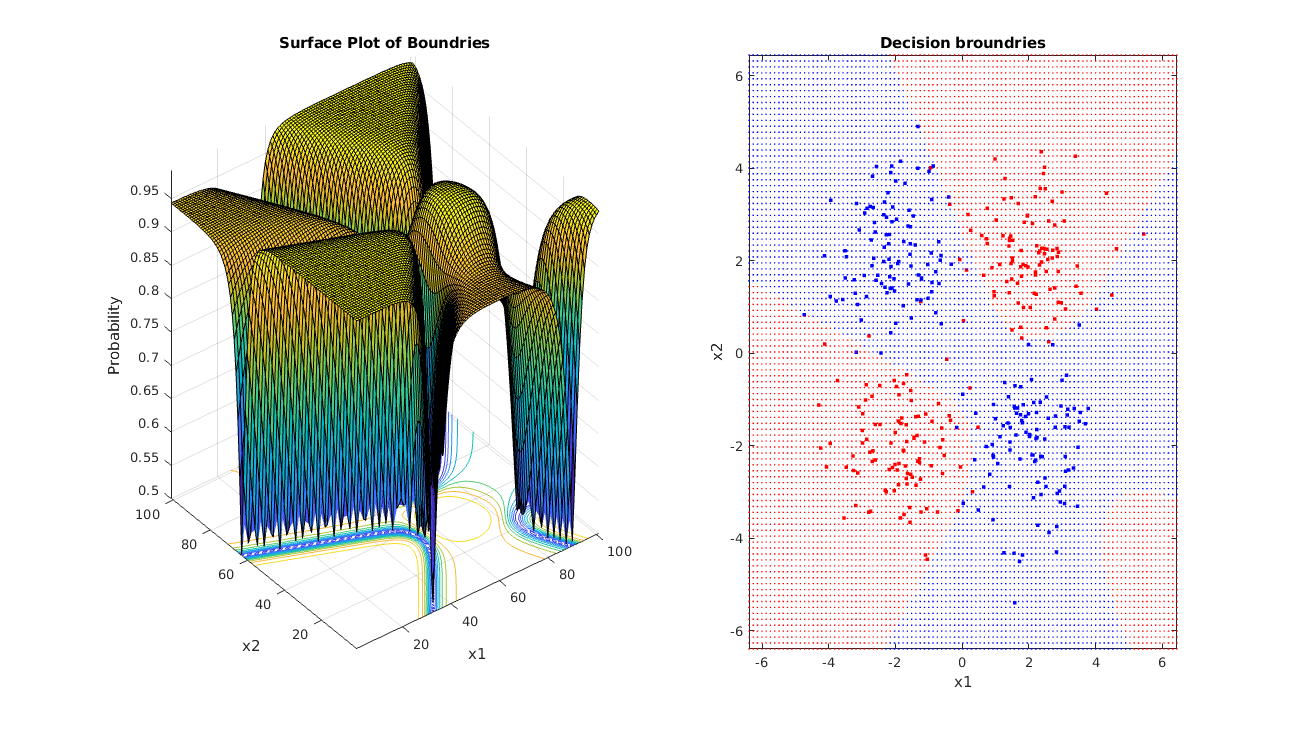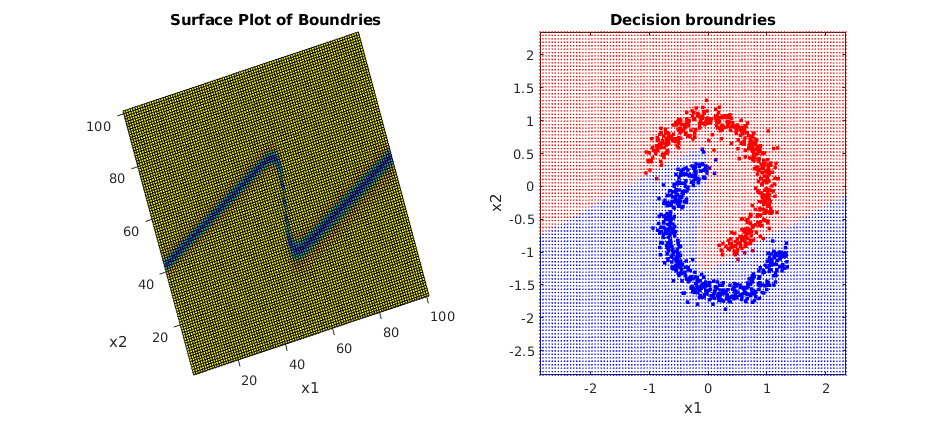4. Kernel Learning & regularization

Kernal Learning

(Linear, Polynomial, Gaussian)

• Linear
• Polynomial
• Gaussian (RBF)

Code and examples with GUI are given here5 Naive Bayes

Probabilistic model

Classifier based on Bayes rule: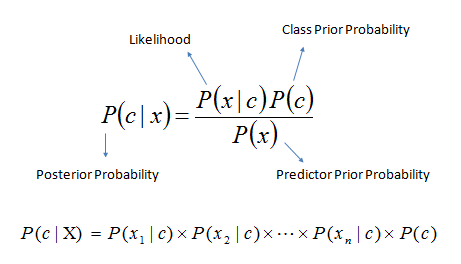Example with jupyter notebook here and Repository

Notebook include example of Iris data, Breast Cancer and Digit classification (MNIST)

Notebook

here is code snippet

``````import numpy as np
import matplotlib.pyplot as plt

# For dataset
from sklearn import datasets
from sklearn.model_selection import train_test_split

# Library provided
from probabilistic import NaiveBayes

X = data.data
y = data.target

Xt,Xs,yt,ys = train_test_split(X,y,test_size=0.3)

print(Xt.shape,yt.shape,Xs.shape,ys.shape)

# Fitting model (estimating the parameters)
clf = NaiveBayes()
clf.fit(Xt,yt)

# Prediction
ytp = clf.predict(Xt)
ysp = clf.predict(Xs)

print('Training Accuracy : ',np.mean(ytp==yt))
print('Testing  Accuracy : ',np.mean(ysp==ys))

print(clf.parameters)

# Visualization
fig = plt.figure(figsize=(12,10))
clf.VizPx()
``````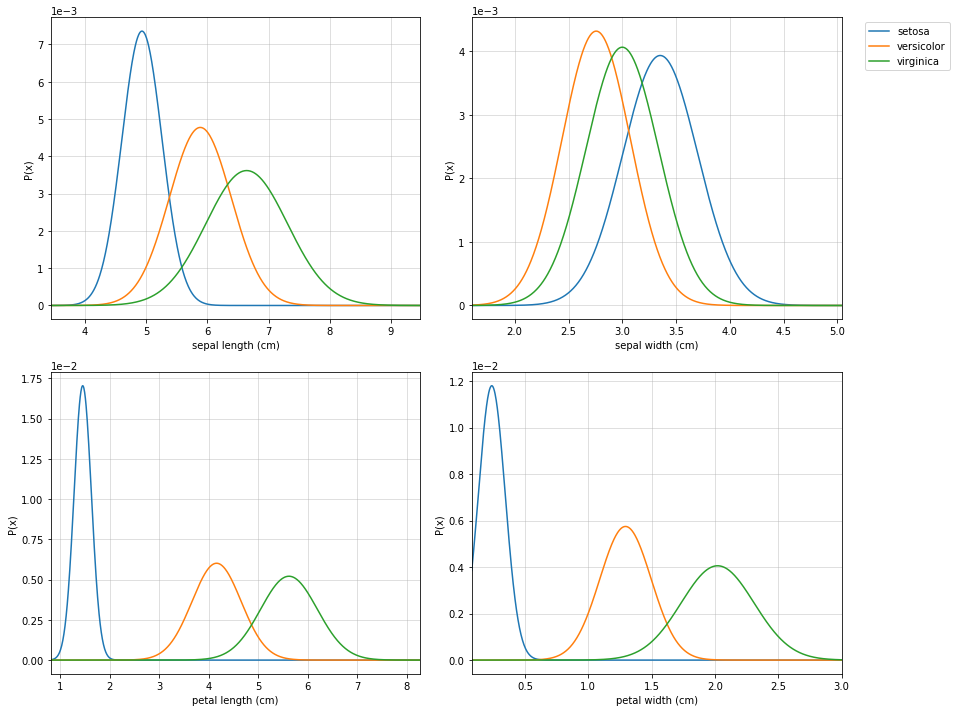6 Decision Trees

Notebook

Import

``````import numpy as np
import matplotlib.pyplot as plt

# Download trees.py and keep in current directory or give a path (if you know how to)
from trees import ClassificationTree, RegressionTree

# For examples
from sklearn import datasets
from sklearn.model_selection import train_test_split
``````

Iris Data

``````data = datasets.load_iris()
X = data.data
y = data.target

feature_names = data.feature_names #Optional
Xt,Xs, yt, ys = train_test_split(X,y,test_size=0.3)
``````

Initiate the classifier and train it

``````clf = ClassificationTree()

# verbose 0 for no progress, 1 for short and 2 for detailed.
# feature_names is you know, else leave it or set it to None

clf.fit(Xt,yt,verbose=2,feature_names=feature_names)
``````

Plot the decision tree

``````# Plot Tree that has been learned
plt.figure(figsize=(15,8))
clf.plotTree(show=True)
``````

Visualizing the tree building while training

Classification: Iris Data, Breast cancer Data Regression::Bostan House price Data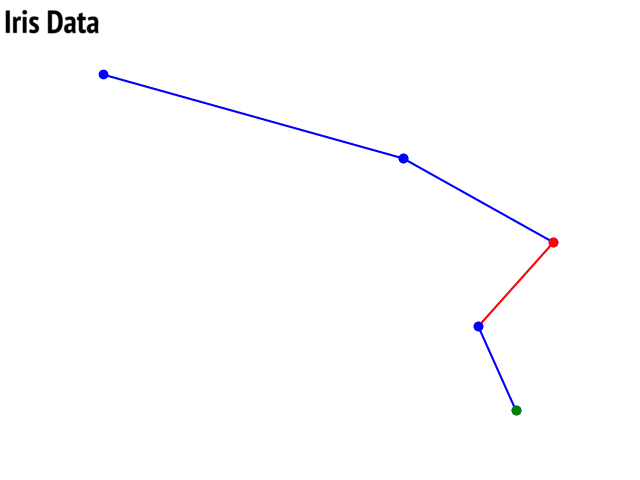Visualization of decision tree after fitting a model

Option to show colored branch: Blue for True and Red for False Or just show all branches as blue with direction to indicate True and False branch

Iris data: Decesion Tree | Cancer data: Decesion Tree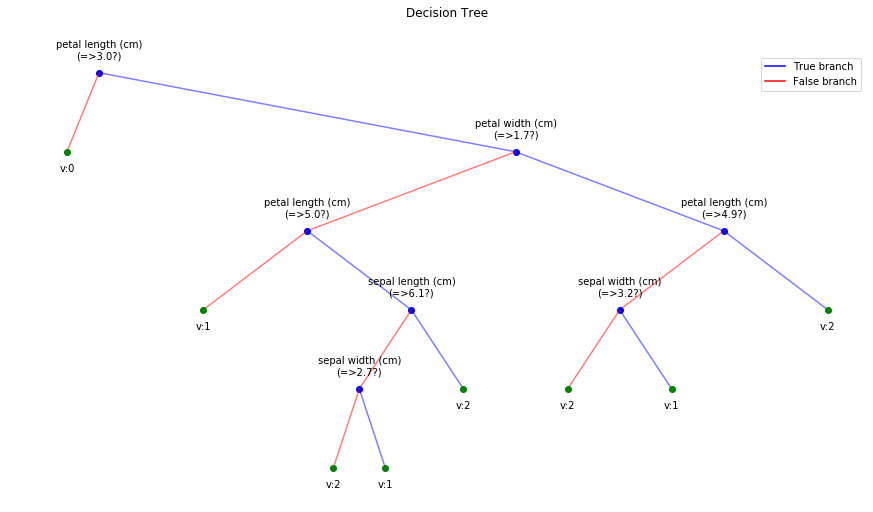|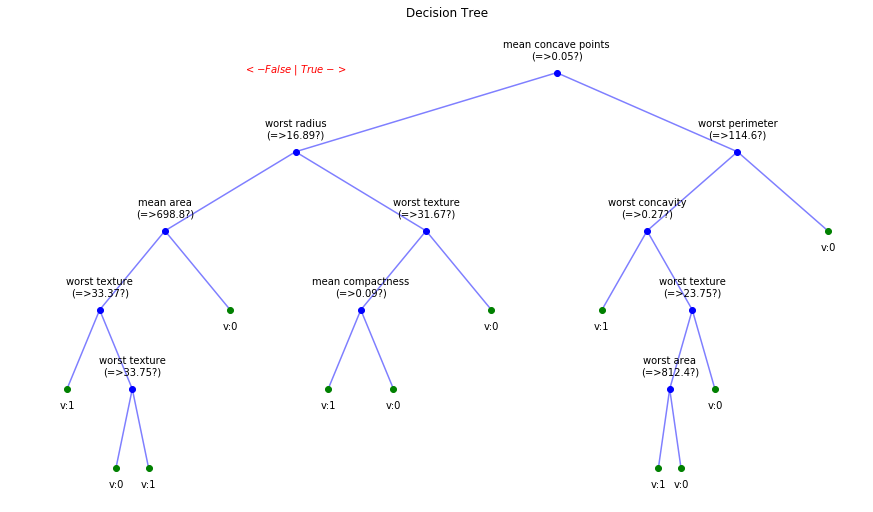Boston data: Decesion Tree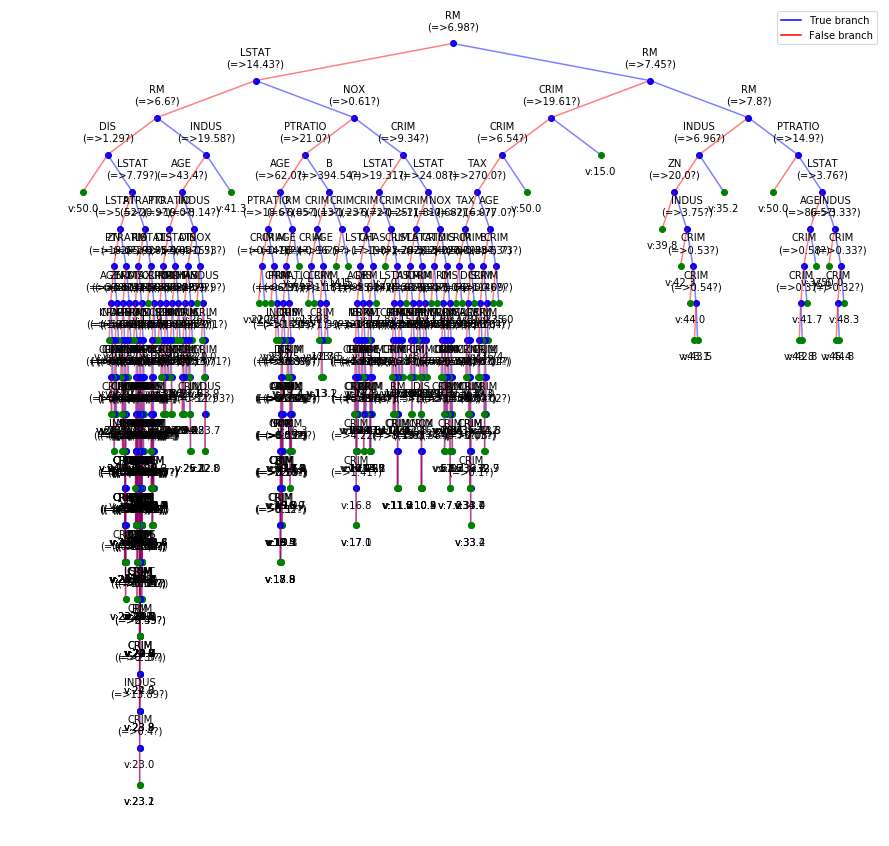Visualizing the progress of tree building while training

Tree building for Cancer Data (Classification)

Detailed view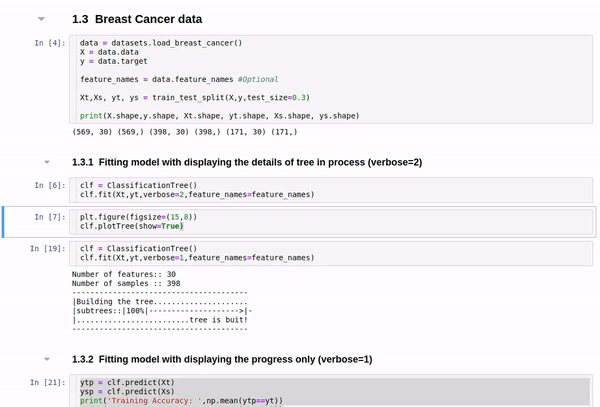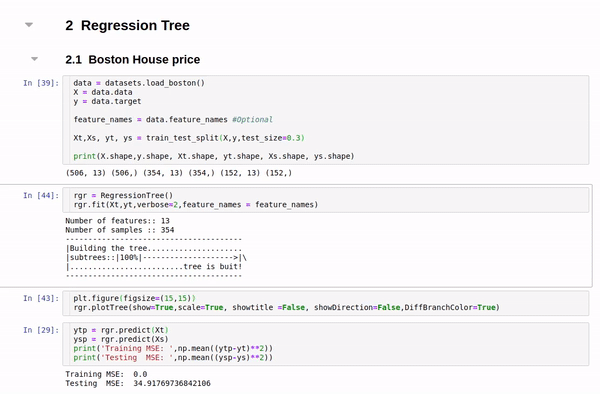Short view